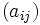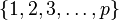# Elementary abelian subgroups of prime-square order in unitriangular matrix group:UT(3,p)

Jump to: navigation, search
This article is about a particular subgroup in a group parametrized by a prime (so we get one group for each prime and a corresponding subgroup for it), up to equivalence of subgroups (i.e., an isomorphism of groups that induces the corresponding isomorphism of subgroups). The subgroup is (generically) elementary abelian group of prime-square order and the group is (generically) prime-cube order group:U(3,p) (see subgroup structure of prime-cube order group:U(3,p)).
The subgroup is a normal subgroup and the quotient group is (generically) group of prime order.
VIEW: Group-subgroup pairs with the same subgroup part | Group-subgroup pairs with the same group part| Group-subgroup pairs with the same quotient part | All pages on prime-parametrized particular subgroups in groups

Let$p$ be an odd prime (The case$p = 2$, where we get Klein four-subgroups of dihedral group:D8 and cyclic maximal subgroup of dihedral group:D8, is somewhat different, though many things are similar).

The group$G = U(3,p)$ is the group of unipotent upper-triangular matrices over the prime field of$p$ elements, and is also the unique (up to isomorphism) non-abelian group of order$p^3$ and exponent$p$. It is defined by the presentation:$G := \langle g,h,k \mid g^p = h^p = k^p = e, gh = hg, gk = kg, khk^{-1} = g^{-1}h \rangle$.

In the matrix description, each matrix$(a_{ij})$ can be described by the three entries$a_{12}, a_{13}, a_{23}$. The matrix looks like:$\begin{pmatrix} 1 & a_{12} & a_{13} \\ 0 & 1 & a_{23}\\ 0 & 0 & 1\end{pmatrix}$

The multiplication of matrices$A = (a_{ij})$ and$B = (b_{ij})$ gives the matrix$C = (c_{ij})$ where:

•$c_{12} = a_{12} + b_{12}$
•$c_{13} = a_{13} + b_{13} + a_{12}b_{23}$
•$c_{23} = a_{23} + b_{23}$

With the matrix description, we can set$g$ as the matrix with$a_{13} = 1$ and the other two entries zero,$h$ as the matrix with$a_{12} = 1$ and the other two entries zero, and$k$ as the matrix with$a_{23} = 1$ and the other two entries zero.

Now define the subgroup:$H_0 := \{ \begin{pmatrix} 1 & a_{12} & a_{13} \\ 0 & 1 & 0\\ 0 & 0 & 1\end{pmatrix} : a_{12}, a_{13} \in \mathbb{F}_p \}$

In addition to this, consider the following$p$ subgroups, parametrized by$\{ 1,2,3,\dots,p \}$:$H_i := \{ \begin{pmatrix} 1 & ia_{23} & a_{13} \\ 0 & 1 & a_{23}\\ 0 & 0 & 1\end{pmatrix} : a_{13},a_{23} \in \mathbb{F}_p \}$

All the$p + 1$ subgroups$H_0, H_1, \dots, H_p$ are elementary abelian subgroups of prime-square order and they are all automorphic subgroups, i.e., they are all related by automorphisms of the whole group.

Note that$H_0$ and$H_p$ in particular are pattern subgroups with respect to the given choice of basis.

## Arithmetic functions

Function Value Explanation
order of whole group$p^3$
order of subgroup$p^2$
index of subgroup$p$
size of conjugacy class of subgroup 1
number of conjugacy classes in automorphism class of subgroup$p + 1$
size of automorphism class of subgroup$p + 1$